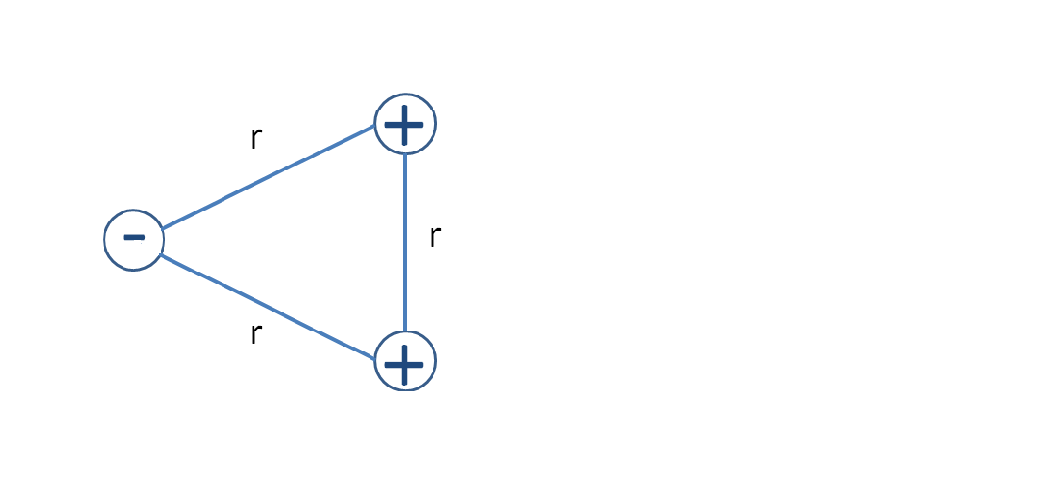# Problem: Three point charges shown below are located at the vertices of an equilateral triangle. If the electric potential energy of two point charges is defined to be zero if the distance between them approaches infinity, the total electric potential energy of the system of those three charges is A) Positive B) Zero C) Negative D) All of the above

###### Problem Details

Three point charges shown below are located at the vertices of an equilateral triangle. If the electric potential energy of two point charges is defined to be zero if the distance between them approaches infinity, the total electric potential energy of the system of those three charges is

A) Positive

B) Zero

C) Negative

D) All of the above